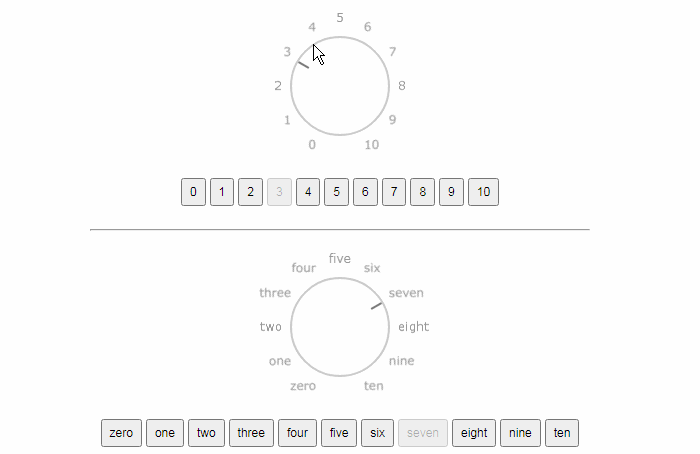/ Knob

# A light weight and intuitive knob component for Vue.js## Vue Knob

A simple, light weight and intuitive knob component for Vue.js.

The vue-knob components attempts to simplify using knobs with your application by providing a simple and customizable control.

Install

Install the package via npm.

``````> npm install @websanova/vue-knob
``````

## Setup

Include the knob component by simply installing it.

``````import Vue from 'vue';

import VueKnob from '@websanova/vue-knob';
Vue.component('el-knob', VueKnob);
``````

The component can then be used as follows.

``````<el-knob
v-model="data.value"
:options="data.options"
/>
``````

## v-model Value

It's important to note that the value returned will be the same value or object set in the `options` prop.

For instance in the below case the value returned to `data.value` will be the original values of `1` or `2`.

``````<el-knob
v-model="data.value"
:options="[1, 2]"
/>
``````

However, if using an object, then entire original object would be returned.

``````<el-knob
:value="data.value"
:options="[{
value: 1,
label: 'one'
}, {
value: 2,
label: 'two'
}]"
@input="data.value = \$event.value"
/>
``````

The reason for this is to allow us to pass through additional parameters with the selected value which will be a common requirement and avoids additional looks ups.

## Manual

If a more manual approach for setting the value is needed use the `value` prop and `@input` event instead of `v-model`.

``````<el-knob
:value="data.value"
:options="data.options"
@input="data.value = \$event.value"
/>
``````

## Size

Adjust the knob size with the `size` prop.

There are five sizes supported out of the box which include `xs`, `sm`, `md`, `lg`, `xl`.

``````<el-knob
v-model="data.value"
:options="data.options"
size="lg"
/>
``````

## Variant

The `variant` prop can be used to quickly set a "theme" class on the knob such as `primary` or `secondary`.

This will simply append a `knob-:variant` class such as `knob-primary`.

``````<el-knob
v-model="data.value"
:options="data.options"
variant="primary"
/>
``````

## Speed

The speed (in seconds) at which the dial turns can also be adjusted using the `min-speed` prop.

Keep in mind that this is a "speed" not a time, so it will be constant regardless of distance.

``````<el-knob
v-model="data.value"
:options="data.options"
:min-speed="5"
/>
``````